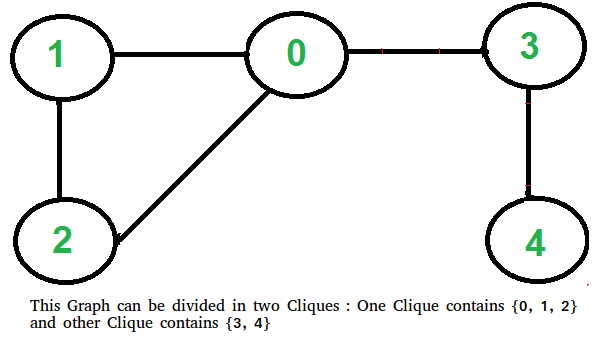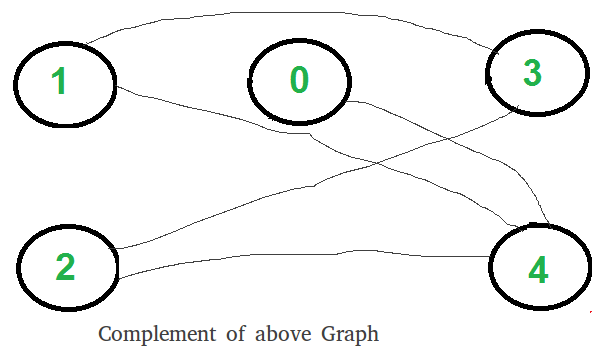Open in App
Not now

# Two Clique Problem (Check if Graph can be divided in two Cliques)

• Difficulty Level : Hard
• Last Updated : 27 Jan, 2023

A Clique is a subgraph of graph such that all vertices in subgraph are completely connected with each other. Given a Graph, find if it can be divided into two Cliques.

Examples:

```Input : G[][] =   {{0, 1, 1, 0, 0},
{1, 0, 1, 1, 0},
{1, 1, 0, 0, 0},
{0, 1, 0, 0, 1},
{0, 0, 0, 1, 0}};
Output : Yes```This problem looks tricky at first, but has a simple and interesting solution. A graph can be divided in two cliques if its complement graph is Bipartitite. So below are two steps to find if graph can be divided in two Cliques or not.

• Find the complement of Graph. Below is the complement graph is above shown graph. In complement, all original edges are removed. And the vertices which did not have an edge between them, now have an edge connecting them.• Return true if complement is Bipartite, else false. The above shown graph is Bipartite. Checking whether a Graph is Bipartite or no is discussed here.

How does this work?
If complement is Bipartite, then graph can be divided into two sets U and V such that there is no edge connecting to vertices of same set. This means in original graph, these sets U and V are completely connected. Hence original graph could be divided in two Cliques.

Implementation:
Below is the implementation of above steps.

## C++

 `// C++ program to find out whether a given graph can be``// converted to two Cliques or not.``#include ``using` `namespace` `std;` `const` `int` `V = 5;` `// This function returns true if subgraph reachable from``// src is Bipartite or not.``bool` `isBipartiteUtil(``int` `G[][V], ``int` `src, ``int` `colorArr[])``{``    ``colorArr[src] = 1;` `    ``// Create a queue (FIFO) of vertex numbers and enqueue``    ``// source vertex for BFS traversal``    ``queue <``int``> q;``    ``q.push(src);` `    ``// Run while there are vertices in queue (Similar to BFS)``    ``while` `(!q.empty())``    ``{``        ``// Dequeue a vertex from queue``        ``int` `u = q.front();``        ``q.pop();` `        ``// Find all non-colored adjacent vertices``        ``for` `(``int` `v = 0; v < V; ++v)``        ``{``            ``// An edge from u to v exists and destination``            ``// v is not colored``            ``if` `(G[u][v] && colorArr[v] == -1)``            ``{``                ``// Assign alternate color to this adjacent``                ``// v of u``                ``colorArr[v] = 1 - colorArr[u];``                ``q.push(v);``            ``}` `            ``// An edge from u to v exists and destination``            ``// v is colored with same color as u``            ``else` `if` `(G[u][v] && colorArr[v] == colorArr[u])``                ``return` `false``;``        ``}``    ``}` `    ``// If we reach here, then all adjacent vertices can``    ``// be colored with alternate color``    ``return` `true``;``}` `// Returns true if a Graph G[][] is Bipartite or not. Note``// that G may not be connected.``bool` `isBipartite(``int` `G[][V])``{``    ``// Create a color array to store colors assigned``    ``// to all vertices. Vertex number is used as index in``    ``// this array. The value '-1' of  colorArr[i]``    ``// is used to indicate that no color is assigned to``    ``// vertex 'i'.  The value 1 is used to indicate first``    ``// color is assigned and value 0 indicates``    ``// second color is assigned.``    ``int` `colorArr[V];``    ``for` `(``int` `i = 0; i < V; ++i)``        ``colorArr[i] = -1;` `    ``// One by one check all not yet colored vertices.``    ``for` `(``int` `i = 0; i < V; i++)``        ``if` `(colorArr[i] == -1)``            ``if` `(isBipartiteUtil(G, i, colorArr) == ``false``)``                ``return` `false``;` `    ``return` `true``;``}` `// Returns true if G can be divided into``// two Cliques, else false.``bool` `canBeDividedinTwoCliques(``int` `G[][V])``{``    ``// Find complement of G[][]``    ``// All values are complemented except``    ``// diagonal ones``    ``int` `GC[V][V];``    ``for` `(``int` `i=0; i

## Java

 `// Java program to find out whether a given graph can be``// converted to two Cliques or not.``import` `java.util.ArrayDeque;``import` `java.util.Deque;``class` `GFG {``static` `int` `V = ``5``;`` ` `// This function returns true if subgraph reachable from``// src is Bipartite or not.``static` `boolean` `isBipartiteUtil(``int` `G[][], ``int` `src, ``int` `colorArr[])``{``    ``colorArr[src] = ``1``;`` ` `    ``// Create a queue (FIFO) of vertex numbers and enqueue``    ``// source vertex for BFS traversal``    ``Deque q = ``new` `ArrayDeque<>();``    ``q.push(src);`` ` `    ``// Run while there are vertices in queue (Similar to BFS)``    ``while` `(!q.isEmpty())``    ``{``        ``// Dequeue a vertex from queue``        ``int` `u = q.peek();``        ``q.pop();`` ` `        ``// Find all non-colored adjacent vertices``        ``for` `(``int` `v = ``0``; v < V; ++v)``        ``{``            ``// An edge from u to v exists and destination``            ``// v is not colored``            ``if` `(G[u][v] == -``1` `&& colorArr[v] == -``1``)``            ``{``                ``// Assign alternate color to this adjacent``                ``// v of u``                ``colorArr[v] = ``1` `- colorArr[u];``                ``q.push(v);``            ``}`` ` `            ``// An edge from u to v exists and destination``            ``// v is colored with same color as u``            ``else` `if` `(G[u][v] == colorArr[u] && colorArr[v] == colorArr[u])``                ``return` `false``;``        ``}``    ``}`` ` `    ``// If we reach here, then all adjacent vertices can``    ``// be colored with alternate color``    ``return` `true``;``}`` ` `// Returns true if a Graph G[][] is Bipartite or not. Note``// that G may not be connected.``static` `boolean` `isBipartite(``int` `G[][])``{``    ``// Create a color array to store colors assigned``    ``// to all vertices. Vertex number is used as index in``    ``// this array. The value '-1' of  colorArr[i]``    ``// is used to indicate that no color is assigned to``    ``// vertex 'i'.  The value 1 is used to indicate first``    ``// color is assigned and value 0 indicates``    ``// second color is assigned.``    ``int` `colorArr[]=``new` `int``[V];``    ``for` `(``int` `i = ``0``; i < V; ++i)``        ``colorArr[i] = -``1``;`` ` `    ``// One by one check all not yet colored vertices.``    ``for` `(``int` `i = ``0``; i < V; i++)``        ``if` `(colorArr[i] == -``1``)``            ``if` `(isBipartiteUtil(G, i, colorArr) == ``false``)``                ``return` `false``;`` ` `    ``return` `true``;``}`` ` `// Returns true if G can be divided into``// two Cliques, else false.``static` `boolean` `canBeDividedinTwoCliques(``int` `G[][])``{``    ``// Find complement of G[][]``    ``// All values are complemented except``    ``// diagonal ones``    ``int` `GC[][]=``new` `int``[V][V];``    ``for` `(``int` `i=``0``; i

## Python3

 `# Python3 program to find out whether a given``# graph can be converted to two Cliques or not.``from` `queue ``import` `Queue` `# This function returns true if subgraph``# reachable from src is Bipartite or not.``def` `isBipartiteUtil(G, src, colorArr):``    ``global` `V``    ``colorArr[src] ``=` `1` `    ``# Create a queue (FIFO) of vertex numbers``    ``# and enqueue source vertex for BFS traversal``    ``q ``=` `Queue()``    ``q.put(src)` `    ``# Run while there are vertices in``    ``# queue (Similar to BFS)``    ``while` `(``not` `q.empty()):``        ` `        ``# Dequeue a vertex from queue``        ``u ``=` `q.get()` `        ``# Find all non-colored adjacent vertices``        ``for` `v ``in` `range``(V):``            ` `            ``# An edge from u to v exists and``            ``# destination v is not colored``            ``if` `(G[u][v] ``and` `colorArr[v] ``=``=` `-``1``):``                ` `                ``# Assign alternate color to this ``                ``# adjacent v of u``                ``colorArr[v] ``=` `1` `-` `colorArr[u]``                ``q.put(v)` `            ``# An edge from u to v exists and destination``            ``# v is colored with same color as u``            ``elif` `(G[u][v] ``and` `colorArr[v] ``=``=` `colorArr[u]):``                ``return` `False` `    ``# If we reach here, then all adjacent``    ``# vertices can be colored with alternate color``    ``return` `True` `# Returns true if a Graph G[][] is Bipartite or not.``# Note that G may not be connected.``def` `isBipartite(G):``    ``global` `V``    ` `    ``# Create a color array to store colors assigned``    ``# to all vertices. Vertex number is used as index ``    ``# in this array. The value '-1' of colorArr[i]``    ``# is used to indicate that no color is assigned``    ``# to vertex 'i'. The value 1 is used to indicate``    ``# first color is assigned and value 0 indicates``    ``# second color is assigned.``    ``colorArr ``=` `[``-``1``] ``*` `V` `    ``# One by one check all not yet``    ``# colored vertices.``    ``for` `i ``in` `range``(V):``        ``if` `(colorArr[i] ``=``=` `-``1``):``            ``if` `(isBipartiteUtil(G, i, colorArr) ``=``=` `False``):``                ``return` `False` `    ``return` `True` `# Returns true if G can be divided into``# two Cliques, else false.``def` `canBeDividedinTwoCliques(G):``    ``global` `V``    ` `    ``# Find complement of G[][]``    ``# All values are complemented``    ``# except diagonal ones``    ``GC ``=` `[[``None``] ``*` `V ``for` `i ``in` `range``(V)]``    ``for` `i ``in` `range``(V):``        ``for` `j ``in` `range``(V):``            ``GC[i][j] ``=` `not` `G[i][j] ``if` `i !``=` `j ``else` `0` `    ``# Return true if complement is ``    ``# Bipartite else false.``    ``return` `isBipartite(GC)` `# Driver Code``V ``=` `5` `G ``=` `[[``0``, ``1``, ``1``, ``1``, ``0``],``     ``[``1``, ``0``, ``1``, ``0``, ``0``],``     ``[``1``, ``1``, ``0``, ``0``, ``0``],``     ``[``0``, ``1``, ``0``, ``0``, ``1``],``     ``[``0``, ``0``, ``0``, ``1``, ``0``]]` `if` `canBeDividedinTwoCliques(G):``    ``print``(``"Yes"``)``else``:``    ``print``(``"No"``)` `# This code is contributed by PranchalK`

## Javascript

 ``

## C#

 `using` `System;``using` `System.Collections.Generic;` `class` `GFG {``    ``static` `int` `V = 5;` `    ``// This function returns true if subgraph reachable from``    ``// src is Bipartite or not.``    ``static` `bool` `IsBipartiteUtil(``int``[, ] G, ``int` `src,``                                ``int``[] colorArr)``    ``{``        ``colorArr[src] = 1;` `        ``// Create a queue (FIFO) of vertex numbers and``        ``// enqueue source vertex for BFS traversal``        ``Queue<``int``> q = ``new` `Queue<``int``>();``        ``q.Enqueue(src);` `        ``// Run while there are vertices in queue (Similar to``        ``// BFS)``        ``while` `(q.Count > 0) {``            ``// Dequeue a vertex from queue``            ``int` `u = q.Dequeue();` `            ``// Find all non-colored adjacent vertices``            ``for` `(``int` `v = 0; v < V; ++v) {``                ``// An edge from u to v exists and``                ``// destination v is not colored``                ``if` `(G[u, v] == -1 && colorArr[v] == -1) {``                    ``// Assign alternate color to this``                    ``// adjacent v of u``                    ``colorArr[v] = 1 - colorArr[u];``                    ``q.Enqueue(v);``                ``}` `                ``// An edge from u to v exists and``                ``// destination v is colored with same color``                ``// as u``                ``else` `if` `(G[u, v] == colorArr[u]``                         ``&& colorArr[v] == colorArr[u])``                    ``return` `false``;``            ``}``        ``}` `        ``// If we reach here, then all adjacent vertices can``        ``// be colored with alternate color``        ``return` `true``;``    ``}` `    ``// Returns true if a Graph G[][] is Bipartite or not.``    ``// Note that G may not be connected.``    ``static` `bool` `IsBipartite(``int``[, ] G)``    ``{``        ``// Create a color array to store colors assigned``        ``// to all vertices. Vertex number is used as index``        ``// in this array. The value '-1' of  colorArr[i] is``        ``// used to indicate that no color is assigned to``        ``// vertex 'i'.  The value 1 is used to indicate``        ``// first color is assigned and value 0 indicates``        ``// second color is assigned.``        ``int``[] colorArr = ``new` `int``[V];``        ``for` `(``int` `i = 0; i < V; ++i)``            ``colorArr[i] = -1;` `        ``// One by one check all not yet colored vertices.``        ``for` `(``int` `i = 0; i < V; i++)``            ``if` `(colorArr[i] == -1)``                ``if` `(!IsBipartiteUtil(G, i, colorArr))``                    ``return` `false``;` `        ``return` `true``;``    ``}` `    ``// Returns true if G can be divided into``    ``// two Cliques, else false.``    ``static` `bool` `CanBeDividedInTwoCliques(``int``[, ] G)``    ``{``        ``// Find complement of G[][]``        ``// All values are complemented except``        ``// diagonal ones` `        ``int``[, ] GC = ``new` `int``[V, V];``        ``for` `(``int` `i = 0; i < V; i++)``            ``for` `(``int` `j = 0; j < V; j++)``                ``GC[i, j] = (i != j) ? -GC[i, j] : 0;` `        ``// Return true if complement is Bipartite``        ``// else false.``        ``return` `IsBipartite(GC);``    ``}` `    ``// Driver program to test above function``    ``static` `void` `Main(``string``[] args)``    ``{``        ``int``[, ] G = { { 0, 1, 1, 1, 0 },``                      ``{ 1, 0, 1, 0, 0 },``                      ``{ 1, 1, 0, 0, 0 },``                      ``{ 0, 1, 0, 0, 1 },``                      ``{ 0, 0, 0, 1, 0 } };` `        ``if` `(CanBeDividedInTwoCliques(G))``            ``Console.WriteLine(``"Yes"``);``        ``else``            ``Console.WriteLine(``"No"``);``    ``}``} ``// this code is cotributed by dany`

Output :

`Yes`

Time complexity of above implementation is O(V2).

This article is contributed by Shubham Saxena. If you like GeeksforGeeks and would like to contribute, you can also write an article using write.geeksforgeeks.org or mail your article to review-team@geeksforgeeks.org. See your article appearing on the GeeksforGeeks main page and help other Geeks.